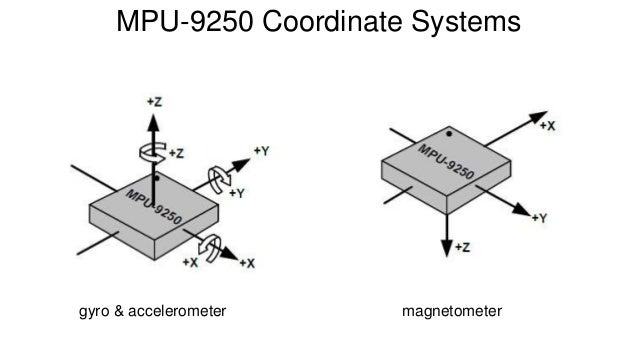Aligning rotation from a imu9250 to a game object

I am experimenting with interfacing unity with real world inputs, specifically I have an imu9250, connected to an arduino clone providing rotation inputs ( either quaternion or pitch/yaw/roll) to unity. (I’m using the DMP functionality of the 9250 to calculate orientation).

The connectivity (over serial for now) works fine, rotating my sensor rotates my game object but I can’t work out how to align my input (programmatically) so that the rotation of the game object rotates in the same direction (in game space).

Any tips/pointers appreciated.

Additional information:My understanding is that the DMP only uses the Gryo and Accelerometer, not the magnetometer.

I can’t find any other references to the coordinate system used outside of the images above. Not mentions in the data sheet as far as I could see http://www.invensense.com/wp-content/uploads/2015/02/PS-MPU-9250A-01-v1.1.pdf

In case anyone comes across this, here is how I solved it (for my needs at least).

This solution requires the target object have an parent GameObject (child position offset 0,0,0). Both must be specified as parameters to the script.

using System.Collections;
using System.Collections.Generic;
using UnityEngine;
using System.IO.Ports;

public class playerController : MonoBehaviour {

public string COMPort = "COM3";
public int baudRate = 115200;

//rotate input - X by 90, Y by 90
private Quaternion offset = new Quaternion();
public Vector3 offsetEuler = new Vector3(90, 90, 0);

public Transform target;
public Transform targetParent;

public bool calibrate = true;
private Quaternion calibrationAngle = new Quaternion();
public bool reverse = false;

SerialPort stream;

void Start () {
stream = new SerialPort(COMPort, baudRate); //Set the port and the baud rate
stream.Open();

// Set the offset
offset = Quaternion.Euler(offsetEuler);
}

private void Update()
{
Quaternion rawInput;
string[] vec3 = value.Split(','); //Arduino returns a Quaternion, note however the order is different to Unity
if (vec3 != "" && vec3 != "" && vec3 != "" && vec3 != "") //Check if all values are recieved
{

rawInput = new Quaternion(float.Parse(vec3), float.Parse(vec3), float.Parse(vec3), float.Parse(vec3));

if (calibrate)
{
// resets the child oritentation so that it remains consistent with world
// needs to be sampled prior to setting parent and set afterwards.
// this could be probably be done cleaner in the Start function
calibrationAngle = target.transform.rotation ;
calibrate = false;
targetParent.transform.rotation = offset * rawInput;
target.transform.rotation = calibrationAngle;
}
targetParent.transform.rotation = offset * rawInput;
}
}
}